﻿ Beyond the Madelung-Klechkowski Rule of <i>aufbau</i> Orbital Filling Principle

### Beyond the Madelung-Klechkowski Rule of aufbau Orbital Filling Principle

Tangour Bahoueddine

World Journal of Chemical Education

## Beyond the Madelung-Klechkowski Rule of aufbau Orbital Filling Principle

Tangour BahoueddineUniversité de Tunis El Manar, Research Unit of Modeling Fundamental Sciences and Didactics, Tunisia

### Abstract

We propose a more general wording for Madelung rule using a new descriptor based on the four quantum numbers n, ℓ, m, ms of the most recently added electron notes K(ZX). By this formulation, K(ZX) will increment as the atomic number Z increases. No exceptions will remain. It can be connected to the first ionization energy.

• Tangour Bahoueddine. Beyond the Madelung-Klechkowski Rule of aufbau Orbital Filling Principle. World Journal of Chemical Education. Vol. 3, No. 6, 2015, pp 160-167. http://pubs.sciepub.com/wjce/3/6/5
• Bahoueddine, Tangour. "Beyond the Madelung-Klechkowski Rule of aufbau Orbital Filling Principle." World Journal of Chemical Education 3.6 (2015): 160-167.
• Bahoueddine, T. (2015). Beyond the Madelung-Klechkowski Rule of aufbau Orbital Filling Principle. World Journal of Chemical Education, 3(6), 160-167.
• Bahoueddine, Tangour. "Beyond the Madelung-Klechkowski Rule of aufbau Orbital Filling Principle." World Journal of Chemical Education 3, no. 6 (2015): 160-167.

 Import into BibTeX Import into EndNote Import into RefMan Import into RefWorks

123
Prev Next

### 1. Introduction

The determination of atomic ground state electronic configuration is a fundamental knowledge and a basic concept for understanding atomic principles of matter. Electronic configuration concept is a consequence of the orbital approximation. Experimental determination of ground state electronic configuration is mainly based on the interpretation of atomic electronic spectra. Some rules can be used to find the electronic configuration of atomic ground state. They are usually named as aufbau (or building-up principle) from the German word meaning "construction". The filling rules are a pillar of the scientific training of students. Their success is partly the consequence of their simple statement, presentation and use. It is obvious that any reader of this paper knows all their details. However those rules remain approximate to the extent that a chemical element in five has an experimental electronic configuration that does not comply with these rules. In reality, as Löwdin  has claimed it, they are empirical in nature and they never been derived from quantum mechanics. The principal rule of aufbau principal was known as the (n+ℓ, n) rule. It consists of two parts: i) For neutral atoms, the electron shells fill up in the order of the quantum number sum (n+ℓ), ii) For electrons having equal values of (n + ℓ), the filling order goes with increasing n.

The authorship of this rule is still debated . Written documents explicitly mention the date 1930 [3, 4] but the periodic table published in 1928 by Janet  was using the sum (n + ℓ) to arrange the atoms into lines. However Goudsmith indicated that Madelung has told to him in a letter in 1926 about this discovery. Thus, it bears the name Madelung’s rule  in Anglo-Saxon countries. But it is known as Klechkowski’s rule in francophone and East European countries since it received its first theoretical justification for his work from 1951 [7, 8, 9]. We will use the two nomenclatures in same time. With Hund's rule [10, 11, 12] and Pauli’s exclusion principle, the Madelung-Klechkowski rule is one of the basic tools for predicting the filling of atomic orbitals.

The theoretical justification of this rule  is more complicated that its common form. Analytical solution to Fermi-Thomas model [15, 16] using Tietz’s approximation  leads to access to the total electrons number of one atom ZX by means of the equation (1)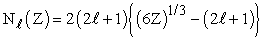(1)

According to the approximate equation (2), the quantum numbers n and ℓ depend on Z.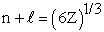(2)

This paper is aimed to propose another empirical formula, easy in use, which enables us to find the true ground state electronic configuration for all atoms. In fact, we will try to give a quantitative expression to the three used rules in the determination of electronic configuration.

### 2. Results and Discussion

Let us begin by analyzing qualitatively the rules that lead to determining the ground state atomic configuration. The concept of electronic configuration is an offshoot of the orbital approximation which considers that it is possible to declare the poly-electrons wave function as a product of one-electron wave functions that are called orbitals. The aufbau orbital filling principle is based on the Madelung-Klechkowski rule that uses the sum of the principal and secondary quantum numbers (n+ℓ) as criterion to select the filling order of sub-shells.

The Madelung-Klechkowski rule applies in two stages: i) For neutral atoms, the electron shells fill up in the order of the quantum number sum (n +). ii) For electrons having equal values of (n + ℓ), the filling order goes with increasing n. This rule is one of those generate a maxi-minimizing operation because it operates in ascending order of the sum (n+ℓ) but minimizes the value of n in case of equal value (n+ℓ). We could merge these two steps by making asymmetric weighting on n and ℓ. We will give a smaller coefficient to ℓ. Simply, we can test a1=1 and a2=0.75 obtaining the term (n +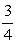ℓ). Between changing a period with ((n+1)+ℓ) and changing a sub-shell according to (n+(ℓ+1)) we have not to choice because we have two different values which are (n+1+0.75 ℓ) and (n+ 0.75 ℓ+0.75). The smallest of them corresponds to the period with the smallest value of n. Figure 1 shows the variation of (n+ℓ) and (n+0.75 ℓ) versus the atomic number Z. Corresponding values are gathered in Table 1.Download asVeiw figureFigures index
Figure 1. Variation of (n+ℓ) and (n+0.75ℓ) versus the atomic number Z

In both cases we are dealing with bearings curves. With the traditional rule, each level is relative to all sub-sells of the same amount (n+ℓ). However, in that we propose, each level corresponds to a different sub-shell and their order totally respect that obtained by conventional application of the Madelung-Klechkowski rule.Download asVeiw figureFigures index
Figure 2. Distribution of quantum number m. In each case the top value (a) corresponds to this work, the bottom (b) the one proposed by Strong  and the top of the box (c) is ms

Let us now deal with Hund' rules. They involve implicitly the magnetic quantum number m forcing sub-shell electrons for distribution on different orbital and explicitly the spin quantum number ms because the electrons are bound to keep up the same spin states. The intervention of the magnetic quantum number is of implicit use because no number is attributed to a specific orbital of the sub-shell. However, some rare periodic tables allow the quantum numbers m and ms at different columns. [18, 19] The attempt is interesting but Strong  attributes orbitals by decreasing m values (Figure 2). For example, with transition elements, he assigns m equal 2 to column 3 then 1 to column 4 and so on decreasing up to -2 to column 7. A second series begins with m equal 2 in column 8 and ends with -2 in the column 12. However, the order changes slightly for lanthanides and actinides starting with m equal 2 instead of 3 (Figure 2). Stowe  did another choice by decreasing mfrom ℓ to – ℓ then he changed the ranging from – ℓ to = ℓ. Our proposition is different because we search a relationship of maximizing the descriptor values. We must start with the smallest value of m. In addition, because m appears as an imaginary quantity in the expression of exact hydrogen atom orbitals, it would be wise to use only its absolute value.

So to differentiate orbitals of the same sub-shell, we propose to add a third contribution versus |m| with weighting coefficient a3. For example, in the sub-shell 3d, orbitals will be ranked in the following order: |m| = 0, 1, 1, 2, 2, 0, 1, 1, 2, 2 as it is shown in Figure 2. By this procedure, the 10 chemical elements of the sub-shell (n-1)d will be separated on 3 groups associated with values of |m| equal 0, 1 and 2. However, this addition has to not change the order of filling sub-shells. We propose for the weighting coefficient a3 the value 0.0075 so that for the greatest |m| value of (n-2)f sub-shell, the contribution is equal to 3* 0.0075 = 0.0275. It remains below 0.75 which is the value between two successive sub-shells.Download asVeiw figureFigures index
Figure 3. Visualization of the partial removing degeneration of the sub-shell orbitals. On bottom, we have the values of (n+0.75ℓ) and above those of (n +0.75ℓ + 0.0075 |m|)

Hund's rule also includes the spin quantum number ms. Strong  attributes the value +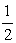to the first half of the sub-shell elements and -the second moiety (Figure 2). Forcing the configuration to maximize its spin multiplicity is in favor of the intervention of S (S=sup (Ms) and Ms=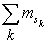)). As the sub-shell is filled by electrons, 2*S will increase to its maximum value equal to the number of orbitals of the sub-shell then decrease by steps of one until his minimum value of zero. We then add a weighting coefficient a4 of 0.005 to reflect this contribution. So we still get a partial degeneration lift as we show in Table 1 for 3d sub-shell which (n+ℓ) is equal to 5. We will have different values between 4.7 and 4.9. They converse according |m| in three groups associated with the values 0, 1 and 2. We see for example, that for each of the four items of value |m| = 1, the spin quantum number input is evaluated by various 0.005 * S with 2*S denoting the number of single electrons that are 2 and 3 and 3 and 2 respectively.

The entire procedure we have just applied is based on the concept of the most recently added electron (MRA). It does not lead to a monotonically increasing trend because the evolution of |m| a sub-shell is that of a sawtooth function and that of the spin quantum number is triangular. However, experimental electronic configuration of chromium or copper shows that the electronic configuration is in fact governed by the stability of the entire valence electrons. That's why we add a fifth contribution equal to the total number of occupied spinorbitales noted So.

#### Table 1. Values of non optimized five contributions intervening in the filling generalized formula for this work as well as the optimized value of the descriptor Ki.

As it is necessary to compensate the behavior of | m | and S that are not monotone increasing, we will choose a higher weighting coefficient a5 equal to 0.025. Thus the maximum contribution associated with spinorbites is 14 * 0.025 = 0.35 remains below the coefficient 0.75 on the quantum number ℓ contribution.

We will therefore propose a formula which generalizes that used to this day, the Madelung-Klechkowski rule. It consists of calculating a real number noted Ki for the ith electron occupying a spinorbital whose expression is given by equation (3):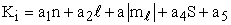(3)

We can also define a similar quantity for the atomic configuration, denoted K (ZX), by summing the N different values of Ki: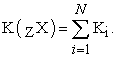We have sought to optimize the values of the parameters ak so as to obtain a monotonic evolution of Ki and K (ZX) as the atomic number Z increases. We obtained the following values by means of the simplex solver: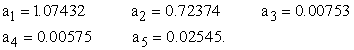For easier use, we will only more simplified numbers: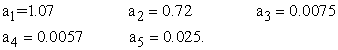Table 2 indicates the calculation details for the two first rows of the standard periodic table.

#### Table 2. Details of the two first rows of the standard periodic table calculation of Ki and K(ZX)Download asVeiw figureFigures index
Figure 4. a) Ki and b) (n+ℓ) versus the atomic number Z

We present in the supplementary materials the calculations details of all chemical elements in the configuration expected by Madelung-Klechkowski rule. In order to compare our results to those obtained by the latter rule, we reported in Figure 4 Ki and (n+ℓ) values versus the atomic number Z.

It becomes clear that the Madelung-Klechkowsk rule is a simplified way to describe the filling process of orbitals. Our proposed formula fits roughly with the following expression Ki versus Z: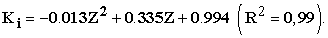Figure 5 depicts the dependence of K(ZX) on Z. We obtain a very good parabolic correlation as: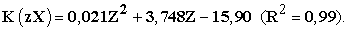Download asVeiw figureFigures index
Figure 5. The variations of K(ZX) versus ZDownload asVeiw figureFigures index
Figure 6. Variations of K(ZX) versus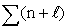values

From Figure 4, we can observe that (n+ℓ) values split each part of Ki roughly in its middle. For a fixed value of (n+ℓ), when Z increases, Ki is lower than it for the first chemical elements and higher for the other. So we obtain a linear correlation between K(ZX) and thevalues as it is shown in Figure 6.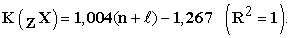Since the proposed formulation incorporates several factors contributing to the stability of the atom in its ground state configuration, we tried to link the value of K(ZX) to the potential of first ionization EI1(ZX).

The challenge is a priori unrealizable because K(ZX) is an increasing function but EI1 is periodic in type. However this periodicity shows that the first value, the alkali one, is low. For the other values, changing EI1 is almost linear. For that, we will treat each period separately by calculating ΔK (ZX), the difference between K (ZX) and the value of the noble gas in the previous period.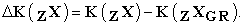We will limit our work to blocks s and p that better generates the notion of periodicity than the nd and nf sub-shells.

We suppose that ΔK(ZX)I is proportional to EI1: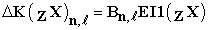#### Table 3. Optimized values of coefficients Bn,ℓobtained by simplex solver

where Bi is a coefficient to optimize so that the sum of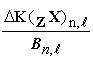is equal to the sum of experimental EI1 (ZX) of each sub-shell: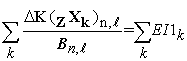where k represents the index relative to an atom of the sub-shell. Table 3 contains the optimized values of coefficients Bn,ℓ obtained by simplex solver.

Figure 7 depicts the values of the first ionization energies and those obtained from our treatment based on the values of K(ZX). We can conclude that our method leads to approximate values of the first ionization energies of the major studied elements.Download asVeiw figureFigures index
Figure 7. ΔK(ZX)n,ℓ and EI1(ZX) versus the atomic number Z

We can find a correlation relationship (Figure 8) between the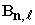coefficients for each type of sub-sell according to the value (n+0.75). For the sub-shells ns, we find the linear relation: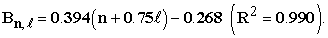For the sub-shells np, the linear relationship based on the value (n + 0.75 ℓ)2 is: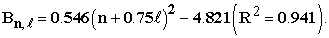We can now propose the approximate expression of Bn,ℓ: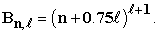Download asVeiw figureFigures index
Figure 8.

### 3. Conclusion

In this work, we established a empirical formula of an descriptor K(ZX) which generalizes the Madelung-Klechkowski rule. It is obtained by taking into account all the electrons from the atom to describe the ground state electronic configuration. K(ZX) is a good descriptor of the atom stability because it is directly related the ionization potential. It is so intuitive to expect that K(ZX) would be able to lead to the exact configuration of all the chemical elements and to overcome all exceptions. Work is in progress concerning the exceptions.

### References

  Leland C. Allen, Eugene T. Knight, The Löwdin challenge: Origin of the n+ℓ, n (Madelung) rule for filling the orbital configurations of the periodic table, Int. J. Quantum Chem.,90(2002) 80-88.In article View Article  Löwdin, P. O. Int. J. Quantum Chem., 3 (1969), 331.In article View Article  V. Karapetoff, J. Franklin Inst. - 210, 609 (1930)In article View Article  Janet, C.: Concordance de l’arrangement quantique de base des électrons planétaires des atomes avec la classification scalariforme. hélicoïdale des éléments chimiques. Beauvais Imprimerie Départementale de l’Oise, Beauvais (1930).In article  Janet C., La classification hélicoïdale des éléments chimiques. Imprimerie Départementale de l’Oise, Beauvais (1928)In article  E. Madelung Die mathematischen Hilfsmittel des Physikers, 3rd ed. Springer, Berlin, 3rd ed., 359, (1936).In article  Klechkowski, V. M., Doklady, , 80(1951), 603.In article  Klechkowski, V. M., Zh. Exsperim. i Teor. Fiz., 23(1952), 115.In article  Klechkowski, V. M., Zh. Exsperim. i Teor. Fiz, 1962 , 41, 465 (Transl. Soviet Physics JETP, 14(1962), 334.In article  F. Hund, Zeitschrift für Physik, 36(1926), 45.In article View Article  F. Hund, Zeitschrift für Physik, 36(1926), 657.In article View Article  F. Hund, Zeitschrift für Physik,42(1927), 93.In article View Article  W. Pauli, “Über den Zusammenhang des Abschlusses der Komplexstruktur der Spektren.” Zeitschrift für Physik, 31(1925), 765-785.In article View Article  ( D. Pan Wong, Theoretical Justification of Madelung's Rule, J. Chem. Edu., 56 (1979) 714-717.In article View Article  Fermi, E., Mem. Accad. Lineei 6(1927), 602.In article  Thomas L. H., Proc. Cambridge Phil. Soe. 23,542 (1927) or Collected Papers, Univ. of Chicago Press, (1962).In article  Tietz, T. J Chem. Phys. 23(1965), 1167.In article View Article  Judith A. Strong, The Periodic Table and Electron Configurations, J. Chem. Edu. 63 (1988) 834-836.In article View Article  Timothy Stowe, the company Instruments Research and Industry (I2R) Inc., (1989).In article